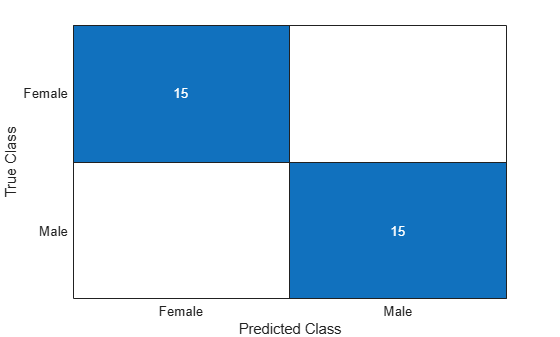# transform

Transform new data using generated features

Since R2021a

## Syntax

``NewTbl = transform(Transformer,Tbl)``
``NewTbl = transform(Transformer,Tbl,Index)``

## Description

example

````NewTbl = transform(Transformer,Tbl)` returns a table with transformed features generated by the `FeatureTransformer` object `Transformer`. The input `Tbl` must contain the required variables, whose data types must match those of the variables originally passed to `gencfeatures` or `genrfeatures` when `Transformer` was created.```

example

````NewTbl = transform(Transformer,Tbl,Index)` returns a subset of the transformed features, where `Index` indicates the features to return.```

## Examples

collapse all

Generate features to train a linear regression model. Compute the cross-validation mean squared error (MSE) of the model by using the `crossval` function.

Load the `patients` data set, and create a table containing the predictor data.

```load patients Tbl = table(Age,Diastolic,Gender,Height,SelfAssessedHealthStatus, ... Smoker,Weight);```

Create a random partition for 5-fold cross-validation.

```rng("default") % For reproducibility of the partition cvp = cvpartition(size(Tbl,1),KFold=5);```

Compute the cross-validation MSE for a linear regression model trained on the original features in `Tbl` and the `Systolic` response variable.

```CVMdl = fitrlinear(Tbl,Systolic,CVPartition=cvp); cvloss = kfoldLoss(CVMdl)```
```cvloss = 45.2594 ```

Create the custom function `myloss` (shown at the end of this example). This function generates 20 features from the training data, and then applies the same training set transformations to the test data. The function then fits a linear regression model to the training data and computes the test set MSE.

Note: If you use the live script file for this example, the `myloss` function is already included at the end of the file. Otherwise, you need to create this function at the end of your .m file or add it as a file on the MATLAB® path.

Compute the cross-validation MSE for a linear model trained on features generated from the predictors in `Tbl`.

`newcvloss = mean(crossval(@myloss,Tbl,Systolic,Partition=cvp))`
```newcvloss = 26.9429 ```
```function testloss = myloss(TrainTbl,trainY,TestTbl,testY) [Transformer,NewTrainTbl] = genrfeatures(TrainTbl,trainY,20); NewTestTbl = transform(Transformer,TestTbl); Mdl = fitrlinear(NewTrainTbl,trainY); testloss = loss(Mdl,NewTestTbl,testY); end```

Train a linear classifier using only the numeric generated features returned by `gencfeatures`.

Load the `patients` data set. Create a table from a subset of the variables.

```load patients Tbl = table(Age,Diastolic,Height,SelfAssessedHealthStatus, ... Smoker,Systolic,Weight,Gender);```

Partition the data into training and test sets. Use approximately 70% of the observations as training data, and 30% of the observations as test data. Partition the data using `cvpartition`.

```rng("default") c = cvpartition(Tbl.Gender,Holdout=0.30); TrainTbl = Tbl(training(c),:); TestTbl = Tbl(test(c),:);```

Use the training data to generate 25 new features. Specify the minimum redundancy maximum relevance (MRMR) feature selection method for selecting new features.

```Transformer = gencfeatures(TrainTbl,"Gender",25, ... FeatureSelectionMethod="mrmr")```
```Transformer = FeatureTransformer with properties: Type: 'classification' TargetLearner: 'linear' NumEngineeredFeatures: 23 NumOriginalFeatures: 2 TotalNumFeatures: 25 ```

Inspect the generated features.

`Info = describe(Transformer)`
```Info=25×4 table Type IsOriginal InputVariables Transformations ___________ __________ ________________________ __________________________________________________________________________________________ zsc(Weight) Numeric true Weight "Standardization with z-score (mean = 153.1571, std = 26.8229)" eb5(Weight) Categorical false Weight "Equal-width binning (number of bins = 5)" c(SelfAssessedHealthStatus) Categorical true SelfAssessedHealthStatus "Variable of type categorical converted from a cell data type" zsc(sqrt(Systolic)) Numeric false Systolic "sqrt( ) -> Standardization with z-score (mean = 11.086, std = 0.29694)" zsc(sin(Systolic)) Numeric false Systolic "sin( ) -> Standardization with z-score (mean = -0.1303, std = 0.72575)" zsc(Systolic./Weight) Numeric false Systolic, Weight "Systolic ./ Weight -> Standardization with z-score (mean = 0.82662, std = 0.14555)" zsc(Age+Weight) Numeric false Age, Weight "Age + Weight -> Standardization with z-score (mean = 191.1143, std = 28.6976)" zsc(Age./Weight) Numeric false Age, Weight "Age ./ Weight -> Standardization with z-score (mean = 0.25424, std = 0.062486)" zsc(Diastolic.*Weight) Numeric false Diastolic, Weight "Diastolic .* Weight -> Standardization with z-score (mean = 12864.6857, std = 2731.1613)" q6(Height) Categorical false Height "Equiprobable binning (number of bins = 6)" zsc(Systolic+Weight) Numeric false Systolic, Weight "Systolic + Weight -> Standardization with z-score (mean = 276.1429, std = 28.7111)" zsc(Diastolic-Weight) Numeric false Diastolic, Weight "Diastolic - Weight -> Standardization with z-score (mean = -69.4286, std = 26.2411)" zsc(Age-Weight) Numeric false Age, Weight "Age - Weight -> Standardization with z-score (mean = -115.2, std = 27.0113)" zsc(Height./Weight) Numeric false Height, Weight "Height ./ Weight -> Standardization with z-score (mean = 0.44797, std = 0.067992)" zsc(Height.*Weight) Numeric false Height, Weight "Height .* Weight -> Standardization with z-score (mean = 10291.0714, std = 2111.9071)" zsc(Diastolic+Weight) Numeric false Diastolic, Weight "Diastolic + Weight -> Standardization with z-score (mean = 236.8857, std = 29.2439)" ⋮ ```

Transform the training and test sets, but retain only the numeric predictors.

```numericIdx = (Info.Type == "Numeric"); NewTrainTbl = transform(Transformer,TrainTbl,numericIdx); NewTestTbl = transform(Transformer,TestTbl,numericIdx);```

Train a linear model using the transformed training data. Visualize the accuracy of the model's test set predictions by using a confusion matrix.

```Mdl = fitclinear(NewTrainTbl,TrainTbl.Gender); testLabels = predict(Mdl,NewTestTbl); confusionchart(TestTbl.Gender,testLabels)```## Input Arguments

collapse all

Feature transformer, specified as a `FeatureTransformer` object.

Features to transform, specified as a table. The rows must correspond to observations, and the columns must correspond to the predictors used to generate the transformed features stored in `Transformer`. You can enter `describe(Transformer).InputVariables` to see the list of features that `Tbl` must contain.

Data Types: `table`

Features to return, specified as a numeric or logical vector indicating the position of the features, or a string array or cell array of character vectors indicating the names of the features.

Example: `1:12`

Data Types: `single` | `double` | `logical` | `string` | `cell`

## Output Arguments

collapse all

Transformed features, returned as a table. Each row corresponds to an observation, and each column corresponds to a generated feature.

## Version History

Introduced in R2021a